# How to choose the optimal wavelength for your biosensor application

July 26, 2017

## To optimize optical biosensors, you need to understand the behavior of light as it impinges upon and travels through the skin.

When visible and infrared waves penetrate human skin, they get absorbed and scattered through the skin layers. The wavelength and properties of each skin layer determine the penetration depth of these waves. By generating absorption and scattering properties as a function of wavelength for each layer of the skin, you can model these properties to figure out the penetration depth of various wavelengths into skin. Then, knowing the penetration depth, you can choose the optimal wavelength for specific biosensor applications.

To optimize optical biosensors, you need to understand the behavior of light as it impinges upon and travels through the skin. Having this knowledge gives you the tools to accurately simulate penetration depth as a function of wavelength. In this article, we’ll look at the absorption and reduced scattering coefficients of human skin layers as a function of wavelength. You can then use these coefficients to simulate penetration depth as a function of wavelength and, ultimately, choose the optimal light source wavelength for a given biosensor application.

# Optical properties of skin layers

Human skin has three main layers from the surface: the blood-free epidermis layer (100 μm thick), the vascularized dermis layer (about 1 mm to 3 mm thick), and subcutaneous adipose tissue (from 1 mm to 6 mm thick, depending on which part of the body). The optical properties of these layers are typically characterized by three factors: absorption (μa) coefficients, scattering (μs) coefficients, and the anisotropy factor (g). The absorption coefficient characterizes the average number of absorption events per unit path length of photons travelling in the tissue. Blood, hemoglobin, b-carotene, and bilirubin are the main absorbers in the visible spectral range. In the IR spectral range, the absorption of water defines the absorption properties of skin dermis. The scattering coefficient characterizes the average number of scattering events per unit path length of photons travelling in the tissue. Finally, the anisotropy factor g represents the average cosine of the scattering angles. Let’s next consider the biological characteristics of each skin layer and how they affect the propagation and absorption of light.

# A closer look at skin structure

The epidermis, the first and outermost section of human skin, can be subdivided into two sublayers: non-living and living epidermis. Non-living epidermis, or stratum corneum (10 μm to 20 μm thick), is composed mainly of dead cells, which are highly keratinized with high lipid and protein content, and has relatively low water content1. In this layer, light absorption is low and relatively uniform in the visible region.

The living epidermis (100 μm thick) propagates and absorbs light. A natural chromophore, melanin2, determines the absorption properties. Melanin comes in two forms: a red/yellow pheomelanin and a brown/black eumelanin which is associated with skin pigmentation. The amount of melanosomes available per unit volume dictates the melanin absorption level. The volume fraction of the epidermis occupied by melanosomes generally varies from 1 percent (lightly pigmented specimens) to 40 percent (darkly pigmented specimens).  The scattering properties of melanin particles depend upon particle size and may be predicted by the Mie theory.

The dermis is a 0.6-mm- to 3-mm-thick structure made up of dense, irregular connective tissue containing nerves and blood vessels. Based on the size of the blood vessels3, the dermis can be divided into two layers. Smaller vessels are closer to the skin surface in the papillary dermis. Larger blood vessels are in the deeper reticular dermis. Absorption in the dermis is defined by the absorption of hemoglobin, water, and lipids. Since oxyhemoglobin and deoxyhemoglobin have different absorption profiles, the oxygen saturation must be known. For an adult, the arterial oxygen saturation is generally higher than 95 percent4. Typical venous oxygen saturation is 60 percent 70 percent5.

The tissue in the dermal layers is rather fibrous, a characteristic that defines the scattering properties of this layer. Light can scatter on interlaced collagen fibrils and bundles as well as single collagen fibrils. The average scattering properties of the skin are dominated by dermal scattering because of the relative thickness of this dermal layer.

The subcutaneous adipose tissue is formed by a collection of fat cells containing stored fat (lipids). Its thickness varies considerably throughout the body: it doesn’t exist in the eyelids, but in the abdomen, it can be up to 6 cm thick. Absorption of hemoglobin, lipids, and water defines absorption of the human adipose tissue. Spherical droplets of lipids, which are uniformly distributed within the fat cells, are the main scatterers of adipose tissue. The diameters of the adipocytes are in the range 15 μm to 250 μm6 and their mean diameter ranges from 50 μm to 120 μm7. Blood capillaries, nerves, and reticular fibrils connecting each cell occupy the spaces between the cells, providing metabolic activity to the fat tissue.

See Figure 1 for a planar five-layer optical model of human skin based on the stratified skin layers we’ve discussed. The model includes the stratum corneum, the living epidermis, the two layers of dermis (papillary and reticular), and the subcutaneous adipose tissue layer. Table 1 presents thickness of the layers as well as typical ranges of blood, water, lipids, and melanin contents; refractive indices of the layers; and mean vessel diameters.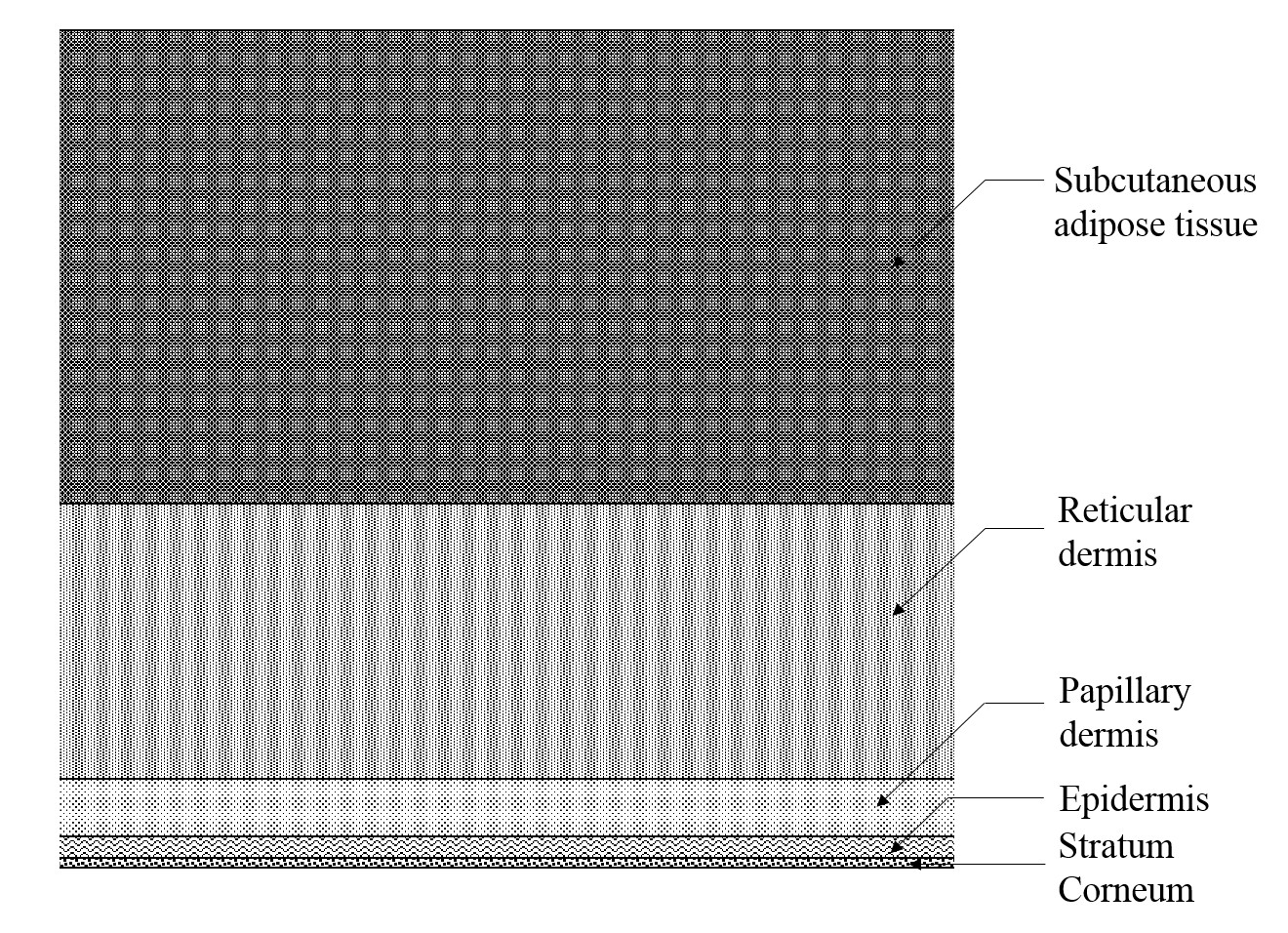[Figure 1 | The five-layer optical model of the skin (not to scale).]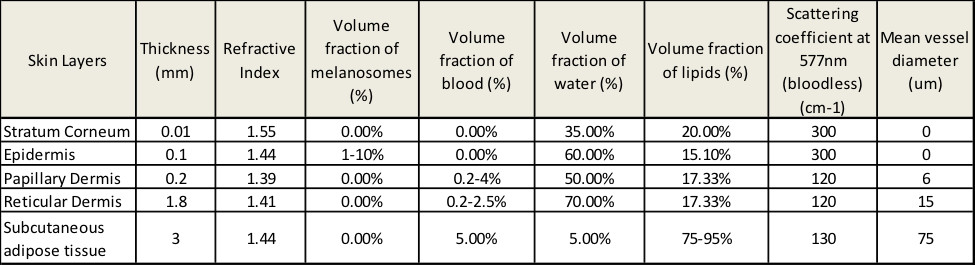[Table 1 | The parameters of skin layers used in the simulation.]

# Absorption coefficients of each skin layer

In the visible and NIR spectral ranges, the absorption coefficient of each layer includes contributions from eumelanin, pheomelanin, oxyhemoglobin, deoxyhemoglobin, bilirubin, β-carotene, lipids, and water. The spectral extinction coefficients for these pigments, denoted eu (λ), ph (λ), ohb (λ), dhb (λ), bil (λ), and β (λ), respectively, are given from the curves shown in Figure 2. The total absorption coefficient for the kth layer is given by:

μak = (ak,eu (λ) + ak,ph (λ)) 𝜗k,mel + (ak,ohb (λ) + ak,dhb (λ) + ak,bil (λ)) 𝜗k,blood
+ (ak,water (λ)) 𝜗k,water + (ak,lip (λ)) 𝜗k,lip
+ (abase (λ) + (ak,β (λ)) (1 − 𝜗k,mel − 𝜗k,blood − 𝜗k,water − 𝜗k,lip)

where = 1,…,5 is the layer number, 𝜗k,mel, 𝜗k,blood, 𝜗k,water, and 𝜗k,lip are the volume fractions of melanin, blood, water, and lipids in the kth layer, and ak,eu (λ), ak,ph (λ), ak,ohb (λ), ak,dhb (λ), ak,bil (λ), ak,water (λ), ak,lip (λ), and ak,β (λ) are the absorption coefficients of eumelanin, pheomelanin, oxyhemoglobin, deoxyhemoglobin, bilirubin, water, lipids, and β-carotene, respectively.  abase (λ) is the wavelength-dependent background tissue absorption coefficient, denoted by 7.84e8 x λ-3.255 cm-1.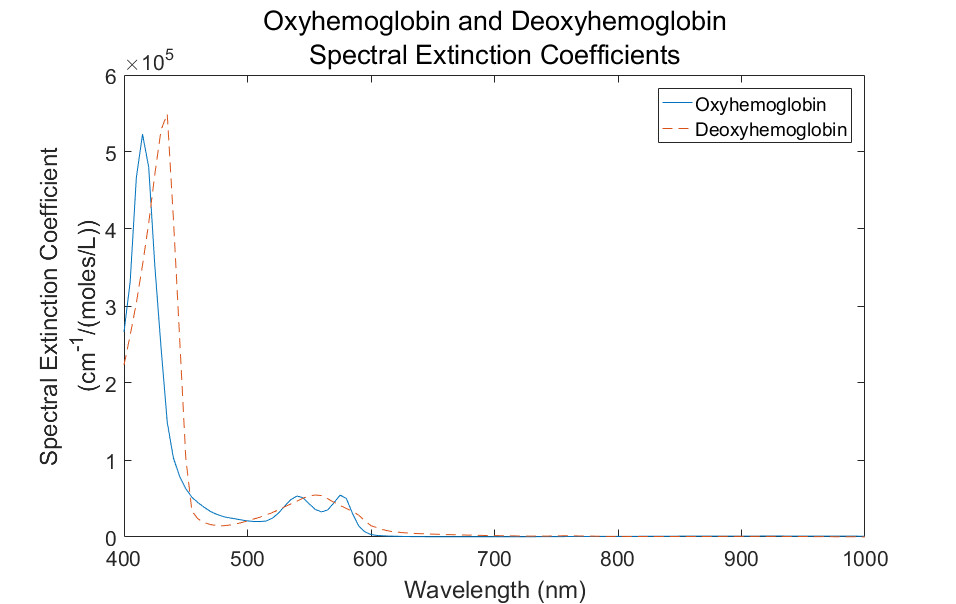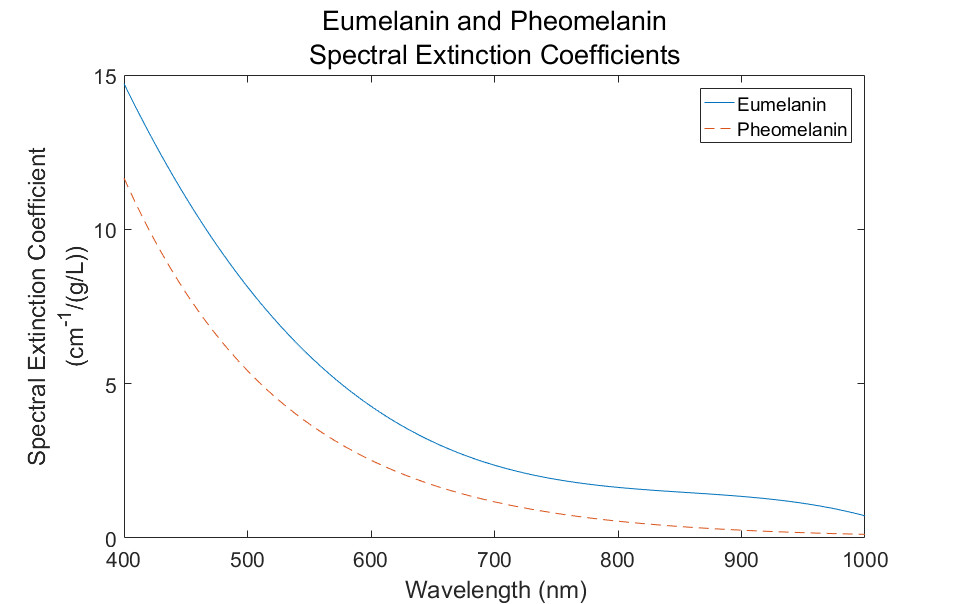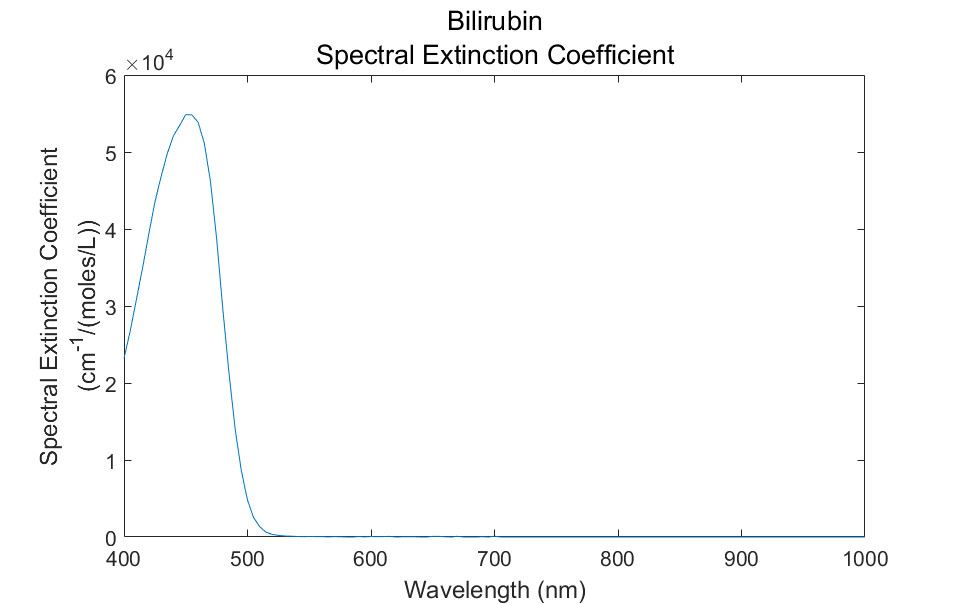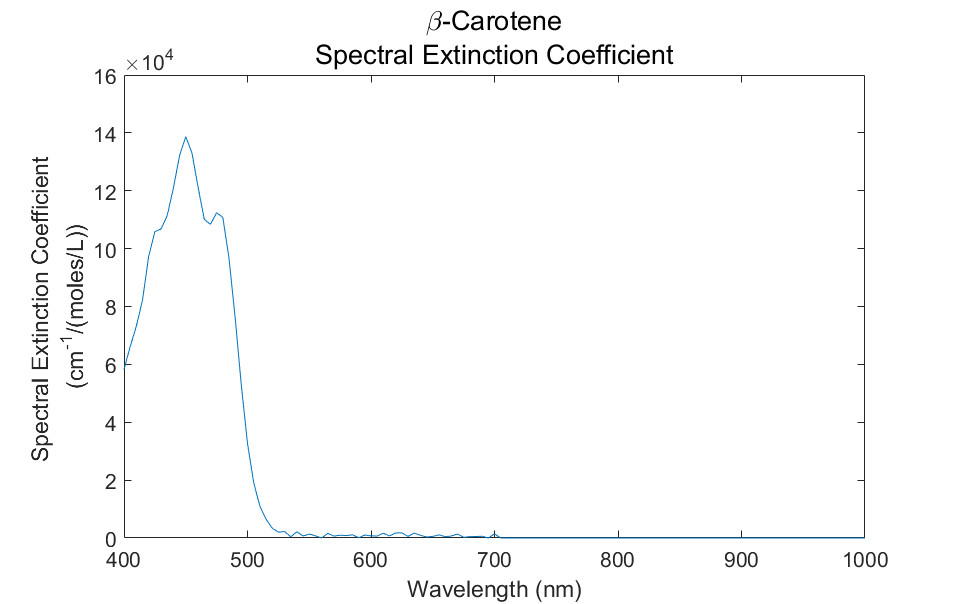[Figure 2 | Spectral extinction coefficient curves for the natural pigments present in skin tissues.]

The eumelanin and pheomelanin absorption coefficients are given by:

ak,eu (λ) = eu (λck,eu and ak,ph (λ) = ph (λck,ph

where ck,eu = eumelanin concentration (g/L) in the kth layer and ck,ph = pheomelanin concentration (g/L) in the kth layer.

The oxyhemoglobin and deoxy hemoglobin absorption coefficients are given by:

aohb (λ) = (ohb (λ) ∕ 66500) ck,hb * γ and ak,dhb (λ) = (dhb (λ) ∕ 66500) ck,hb * (1 γ)

where 66500 = molecular weight of hemoglobin (g/mol), ck,hb = hemoglobin concentration of the blood (g/L) in the kth layer,and γ = ratio of oxyhemoglobin to the total hemoglobin concentration.

The absorption coefficient of bilirubin is given by:

ak,bil (λ) = (bil (λ) ∕ 585) ck,bil

where 585 = molecular weight of bilirubin (g/mol) and ck,bil = bilirubin concentration (g/L) in the kth layer.

The β-carotene absorption coefficient ak,β (λ) is given by:

ak,β (λ= (β (λ) ∕ 537) ck,β

where 537 = molecular weight of β-carotene (g/mol) and ck,β = β-carotene concentration (g/L) in the kth layer.

The absorption coefficient of water is given by:

ak,water (λ= water (λck,water

where ck,water = water concentration (g/L) in the kth layer.

The lipid absorption coefficient is given by:

ak,lip (λlip (λck,lip

where ck,lip = lipid concentration (g/L) in the kth layer.

# Scattering coefficient

The total scattering coefficient for the kth layer can be defined as:

μsk = 𝜗k,blood Cμsblood (λ) + (1 − 𝜗k,bloodμsT(λ

where Ck is a correction factor that is defined by the mean vessel diameter. The blood scattering coefficient as a function of wavelength and μsTk define the total scattering coefficient of the bloodless tissue layer.

The following relations can be used for Ck8:

C= 1/(1+ a (0.5 μsblood dk,vessels)b)

where dk,vessels is the blood vessel’s diameter (cm) in the kth layer. In the case of collimated illumination of the vessels, the coefficients a and b have values a = 1.007 and b = 1.228. In the case of diffuse illumination of the vessels, the coefficients a and b have values a = 1.482 and b = 1.151.

The total scattering coefficient of the bloodless tissue is given by9:

μsTk (λ) = μs0k (577nm / λ)

where μs0are the scattering coefficients at the reference wavelength 577nm listed in Table 1. Note: μsTk falls monotonically with the increase in the wavelength.

The expression for the anisotropy of scattering may be constructed to include the contribution from blood9:

gk (λ) = (𝜗k,blood Cμsblood (λ) gblood + (1 − 𝜗k,bloodμsT(λ) gT (λ))/ μsk (λ)

where gT (λ) is the anisotropy factor of the bloodless tissue and

gk (λ) = 0.7645 + 0.2355 [1– exp ((– λ – 500nm)/729.1nm)]

Finally, the reduced scattering coefficients is defined as μs'(λ) = μsk (λ)(1 – g(λ)).

# Applying computer simulations to determine penetration depth

Zemax Optics Studio software was used to determine penetration depth as a function of wavelength. The software uses a Monte Carlo (MC) method to trace optical rays propagating in complex inhomogeneous, randomly scattering, and absorbing media. To perform basic MC modeling of an individual photon packet’s trajectory, we can apply the following sequence of elementary simulations: photon path length generation, scattering and absorption events, reflection and/or refraction on the medium boundaries. Scattering events can be characterized by the Henyey-Greenstein phase function fHG (θ), which describes the new photon packet’s direction after scattering:

fHG (θ) = (1/4π)((1– g2)/(1 + g– 2gcosθ)3/2

where θ is the polar scattering angle. The distribution over the azimuthal scattering angle was assumed as uniform. The specular reflection from the air-tissue surface is also considered in the simulations.

Using this MC methodology requires that you have the values of absorption, as well as the scattering coefficients and anisotropy factor of each skin layer, its thickness, and refractive index. You will also need to have the mean path defined as the inverse of the scattering coefficient.

# Results

Using the optical properties we’ve discussed with the Henyey-Greenstein scattering phase function and Zemax optical software, we can simulate any biosensor configuration and determine the maximum penetration depth as a function of wavelength. As a use case, consider the following typical LED-photodiode (PD) biosensor configuration (Table 2 and Figure 3) and skin properties shown in Table 3. We performed a simulation to determine the maximum penetration depth as a function of wavelength.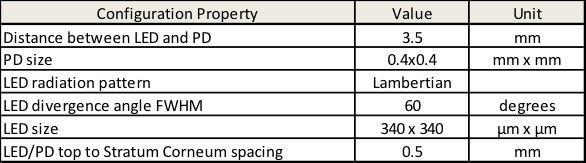[Table 2 | Biosensor configuration used in simulation.]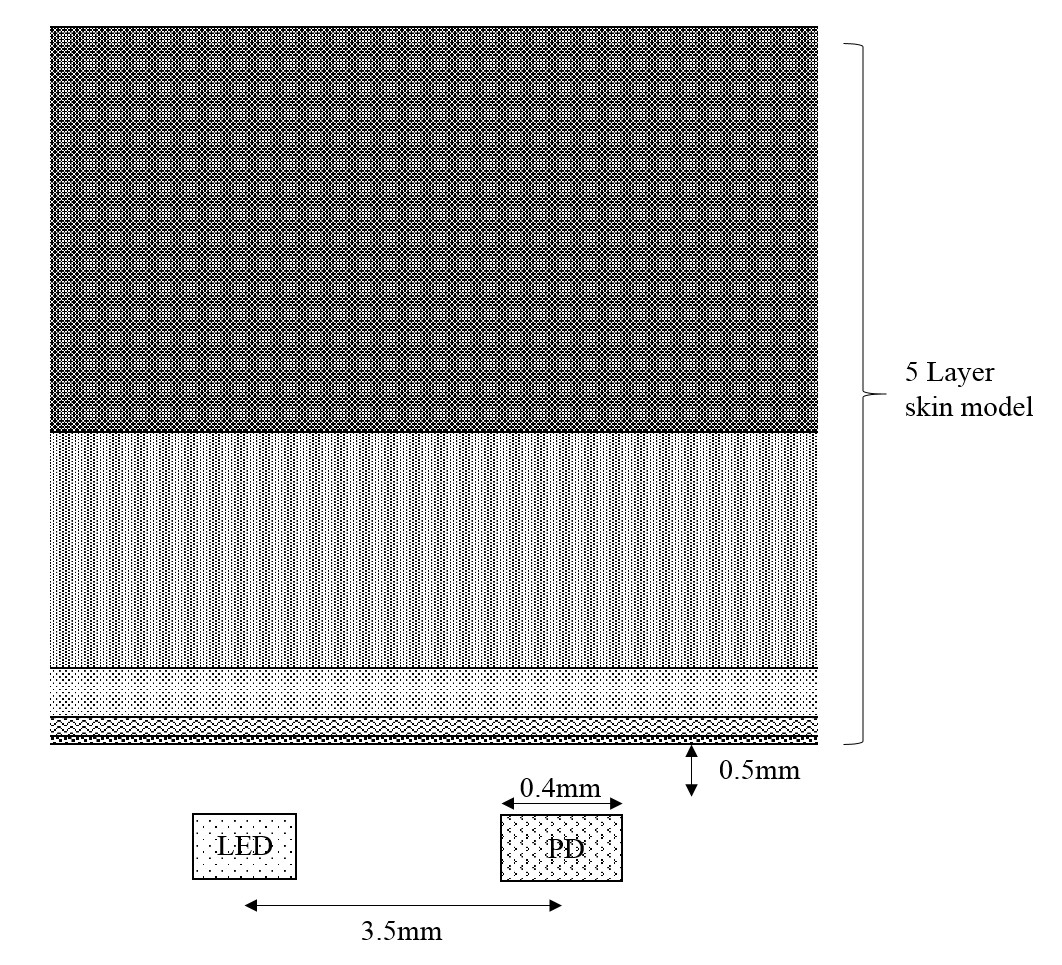[Figure 3 | Dimensions of biosensor configuration used in simulation.]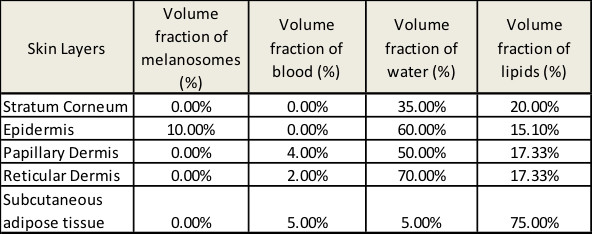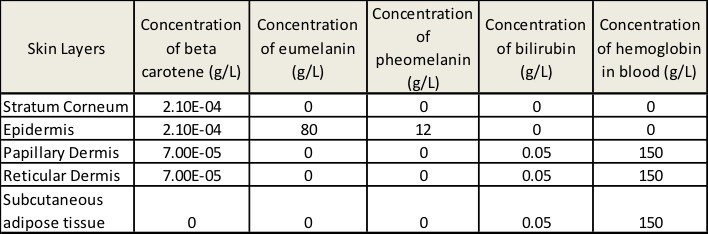[Table 3 | Skin properties used in simulation.]

The absorption coefficients of the skin layers were calculated based on the presented optical model, as shown in Figure 4.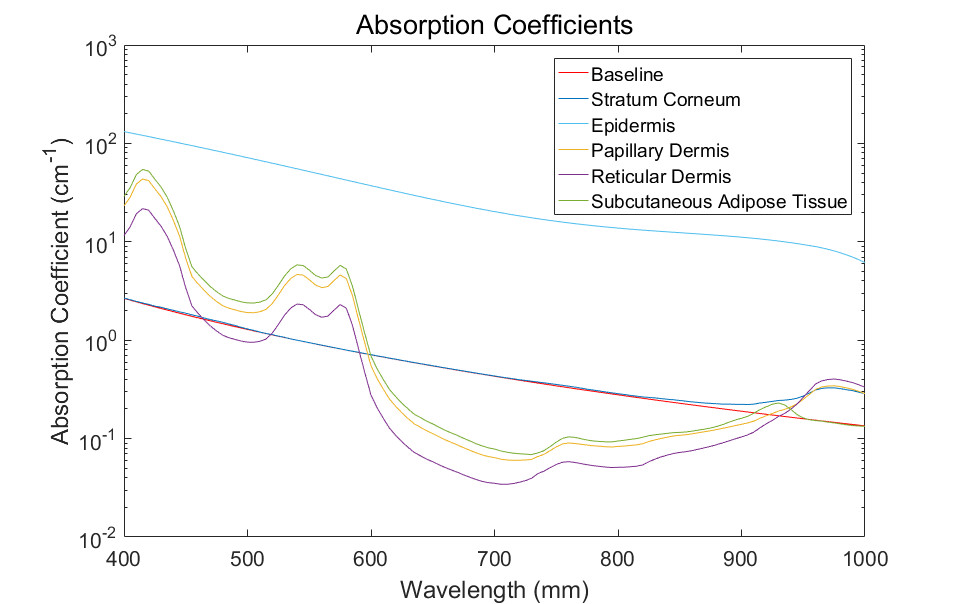[Figure 4 | Absorption spectra of different skin layers calculated based on the presented optical model.]

The scattering coefficient, anisotropy factor, and mean path of the skin layers have been calculated using the presented model, with the result presented in Figures 5-7.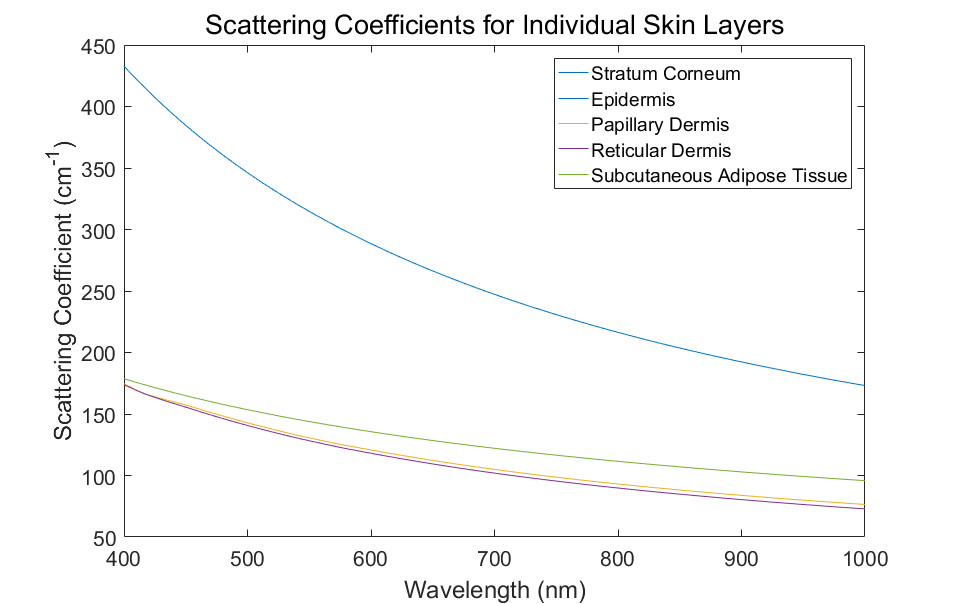[Figure 5 | Scattering coefficient of different skin layers calculated using the presented optical model.]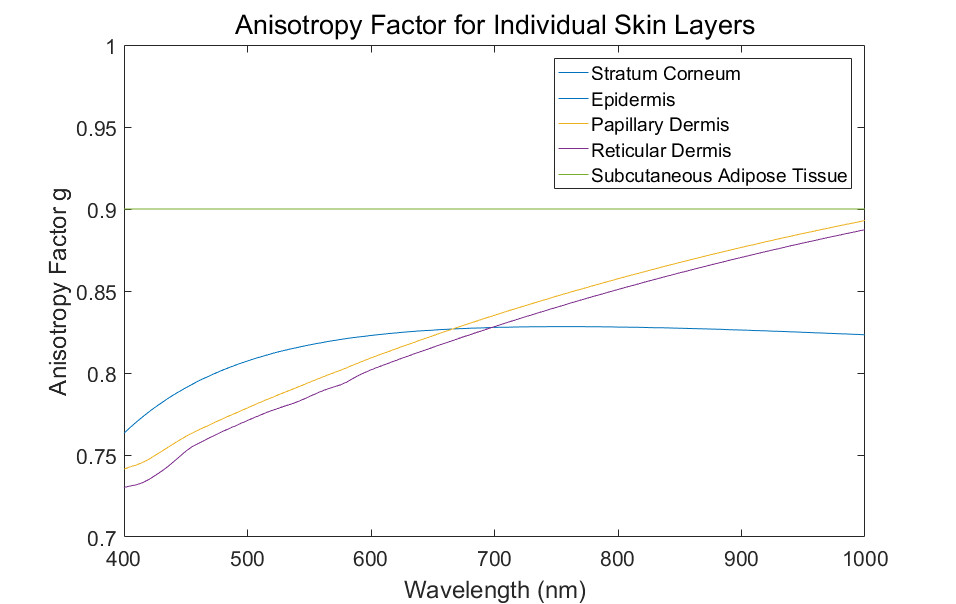[Figure 6 | Anisotropy factor of different skin layers calculated according to the presented optical model.]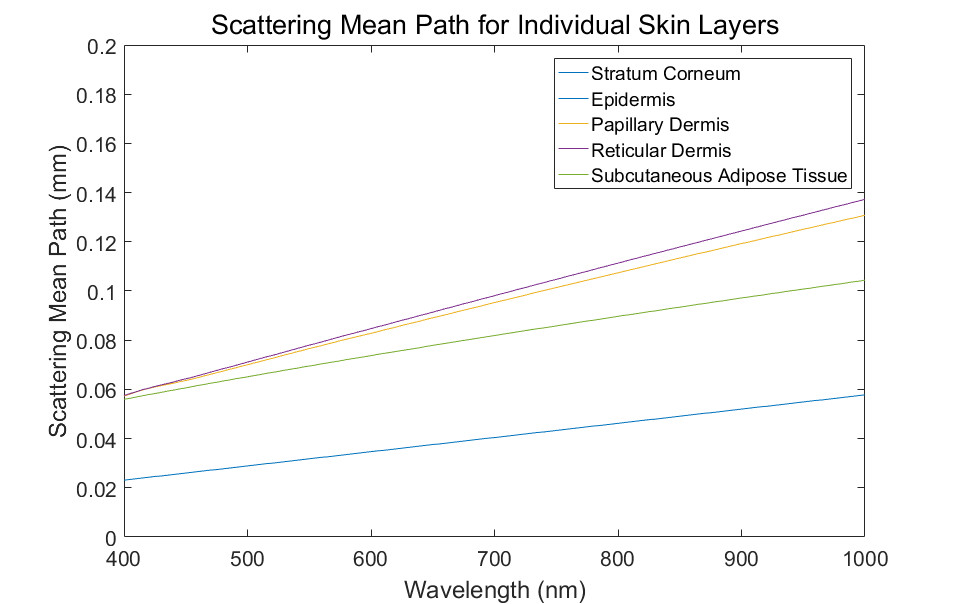[Figure 7 | Scattering mean path of different skin layers calculated using the presented optical model.]

To determine the performance of a biosensor, it’s essential to consider the penetration depth of light into a biological tissue. Using the absorption and reduced scattering coefficient values presented earlier in this article, we simulated optical penetration depth, presenting the results in Figure 8.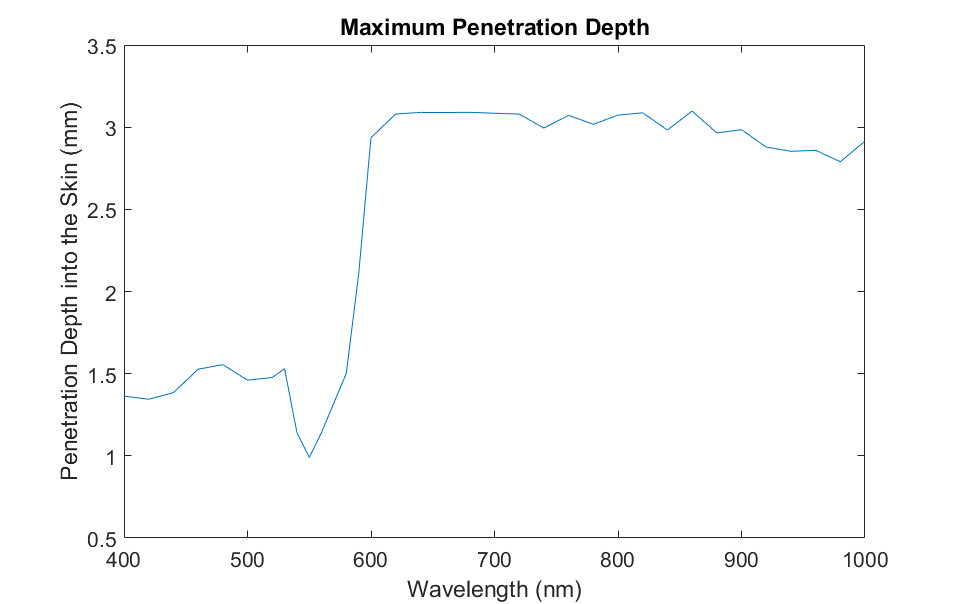[Figure 8 | Simulated maximum penetration depth for the situation shown in Figure 3 and Table 3.]

# Conclusions

In this article, we have modeled human skin tissue according to a five-layer structure, with each layer representing its corresponding anatomical layer. To simulate light tissue interaction, we modeled the biological characteristics of each layer with three wavelength-dependent numbers, absorption coefficient, scattering coefficient, and anisotropy factor. We used a commercial ray-tracing software to calculate penetration depth of light into skin tissue to simulate the performance of optical biosensor architectures.

### References

1. K. S. Stenn, "The skin, " Cell and Tissue Biology ed. by L. Weiss, Baltimore: Urban & Shwarzenberg, 541-572 (1988).
2. M. R. Chedekel, "Photophysics and Photochemistry of Melanin, " In: L. Zeise, M. R. Chedekel and T. B. Fitzpatrick, Eds., Melanin: Its Role in Human Photoprotection, Valdenmar, Overland Park, pp. 11-22 (1994).
3. T. J. Ryan, "Cutaneous Circulation," in Physiology, Biochemistry, and Molecular Biology of the Skin, 1, ed. by L.A. Goldsmith, Oxford University Press, Oxford (1991).
4. A. Zourabian, A. Siegel, B, Chance, N. Ramanujan, M. Rode and D. A. Boas, "Trans-abdominal monitoring of fetal arterial blood oxygenation using pulse oximetry, " J. Biomed. Opt. 5 391–405 (2000).
5. T. Hamaoka et al, "Quantification of ischemic muscle deoxygenation by near infrared time-resolved spectroscopy, " J. Biomed. Opt. 5 102–5 (2000).
6. M. I. Gurr, R. T. Jung, M. P. Robinson and W. P. T. James, "Adipose tissue cellularity in man: the relationship between fat cell size and number, the mass and distribution of body fat and the history of weight gain and loss," Int. J. Obesity 6 419–36 (1982).
7. Y. Taton "Obesity, Pathphysiology, Diagnostics, Therapy", Warsaw: Medical Press (1981).
8. W. Verkruysse, G. W. Lucassen, J. F. de Boer, D. J. Smithies, J. S. Nelson, M. J. C. van Gemert, "Modelling light distributions of homogeneous versus discrete absorbers in light irradiated turbid media," Phys. Med. Biol. 42, 51-65 (1997).
1. G. Altshuler, M. Smirnov, I. Yaroslavsky, "Lattice of optical islets: a novel treatment modality in photomedicine," Journal of Physics D: Applied Physics 38, 2732-2747 (2005).

Judy Hermann is the Principal Member of Technical Staff, Applications, Industrial and Healthcare business unit at Maxim Integrated.